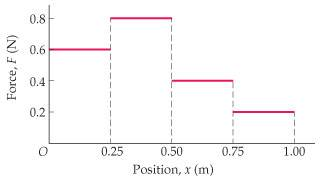# What is the final position of the object?

## Homework Statement

What is the final position of the object if its initial position is x = 0.40 m and the work done on it is equal to -0.19 J?work(J)= Fx ΔX

## The Attempt at a Solution

I do not know where to begin??? please give me a hint where to begin.

haruspex
Homework Helper
Gold Member
2020 Award
It starts at 0.4m. What strength of force acts on it there, and in which direction?
If it were then moved by the force to 0.5m, how much work has the force done?

It starts at 0.4m. What strength of force acts on it there, and in which direction?
If it were then moved by the force to 0.5m, how much work has the force done?
work would be .08 J in positive direction

haruspex
Homework Helper
Gold Member
2020 Award
work would be .08 J in positive direction
Right. Is that going in the right direction to reach a work done of -0.19 J?

Right. Is that going in the right direction to reach a work done of -0.19 J?

No it would not. You would have to go to the left side.

haruspex
Homework Helper
Gold Member
2020 Award
No it would not. You would have to go to the left side.
Right, so go to the left, but stop when you get to a change in the force and see how the total work is going.

Right, so go to the left, but stop when you get to a change in the force and see how the total work is going.

I believe it is either between .12m or .11 m? Am i right?

haruspex
Homework Helper
Gold Member
2020 Award
I believe it is either between .12m or .11 m? Am i right?
Do you mean between x=.11m and x=.12m, or between .11m and .12m left of the 0.25m mark?

Do you mean between x=.11m and x=.12m, or between .11m and .12m left of the 0.25m mark?

from x=.40m to x=.25m work is= .12J
from x=.25m to x=.13m work is = .072 j

total work =.192 J. since I am working backwards I am assuming the answer is negative.

Therefore, my final position would be .13 m?

haruspex
Homework Helper
Gold Member
2020 Award
from x=.40m to x=.25m work is= .12J
from x=.25m to x=.13m work is = .072 j

total work =.192 J. since I am working backwards I am assuming the answer is negative.

Therefore, my final position would be .13 m?
Yes.

Yes.

Thank you for the help!! :)